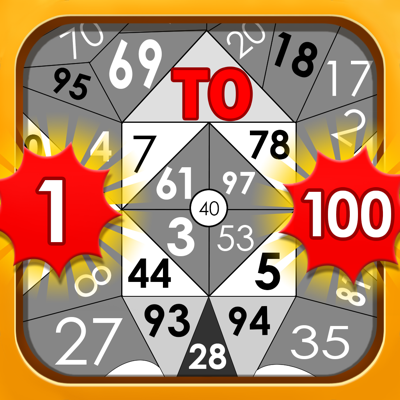# Find The Numbers 1 to 100Find The Difference,Hidden No.

1444134054Category
Games & Puzzle & Board
Current version
1.3
Price
Free
Size
28 MB
Last Update
Aug 29, 2019
Platform
iOS

See more data

## User  Reviews for Find The Numbers 1 to 100

Reviews 5 Avg. Rating 4.6Why to reply to reviews?

User reviews affect conversion to installs and app rating. Featured and helpful reviews are the first to be noticed by users and in case of no response can affect download rate. This is why it is highly recommended to reply to them.

## About Find The Numbers 1 to 100

Developed by the Mrudul Babariya,

who have also released the following apps .

Find The Numbers 1 to 100 has 5 user reviews.

Find The Numbers 1 to 100 has an average rating of 4.6.

The latest version of Find The Numbers 1 to 100 (1.3) ​was ​released on Aug 29, 2019.

You can download Find The Numbers 1 to 100 ​here: 1444134054 .

## Find The Numbers 1 to 100 Description 3026 chars

------------
Find the number from 1 to 100 is a addictive game for number and alphabet finder.
All the number form 1 to 100 generate randomly.
You need to find number as per instruction given on top middle.
More than 50 different levels in the game.
There are 6 different types of board in the game.

Mode
-----
1) Relaxed mode.
2) Timed mode.

Levels
-------
1)Number
—————
Find the number from 1 to 10
Find the number from 11 to 25
Find the

number from 26 to 45
Find the number from 55 to 46
Find the number from 75 to 56
Find the number from 100 to 76

Find the even number from 1 to 40
Find the odd number from 1 to 40
Find the even number from 100 to 50
Find the odd number from 100 to 50

Find the number from 1 to 60 which is divide by 3
Find the number from 60 to 1 which is divide by 3

Find the number from 1 to 80 which is divide by 4
Find the number from 80 to 1 which is divide by 4

Find the number from 1 to 100 which is divide by 5
Find the number from 100 to 1 which is divide by 5

Find the number from 100 to 1 which is divide by 4
Find the number from 100 to 1 which is divide by 3

Find the number from 1 to 50
Find the number from 51 to 100
Find the number from 100 to 1

2)Alphabets
——————
Find the alphabet from A to M
Find the alphabet from N to Z
Find the alphabet from a to m
Find the alphabet from n to z
Find the alphabet from A to Z
Find the alphabet from a to z
Find the even alphabet from A a , B b , ..... , M m
Find the even alphabet from N n , O o , ..... , Z z

Find the alphabet from M to A
Find the alphabet from Z to N
Find the alphabet from m to a
Find the alphabet from z to n
Find the alphabet from Z to A
Find the alphabet from z to a
Find the even alphabet from M m , L l , ..... , A a
Find the even alphabet from Z z , Y y , ..... , N n

3)Hidden Number
————————
You need to find the numbers which is in upper panel.

4)Find the Difference / Spot the Difference
————————————————————
Find the 10 differences form both pictures.

How to Play ?
Tap on number/alphabet as per instruction.
There is a hint functionality so if you can’t find number/alphabet use it.

Who can play ?
No age limit.

Game Features
Realistic graphics and ambient sound.
Realistic stunning and amazing animations.
Real-time particles & effects
Smooth and simple controls.
User friendly interface and interactive graphics.

Important Point
If you getting stuck you can use hint to find number/alphabet.

Benefits
Improve focus.
Improve IQ.